# Detecting A Single Frequency Efficiently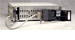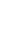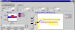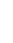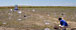## Introduction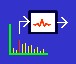There are various ways to detect the presence of a special known frequency in a monitored signal. A simplistic way is to take an FFT (Fast Fourier Transform) of the signal and check whether the desired frequency is present. That is not very efficient, however, because most of the computed results are ignored. A discrete Fourier transform (DFT) produces the same numerical result for a single frequency of interest, making it a better choice for tone detection. The Goertzel Algorithm is a DFT in disguise, with some numerical tricks to eliminate complex number arithmetic, roughly doubling the efficiency. This note presents the Goertzel Algorithm [1,2], and in particular, ways to improve its ability to isolate frequencies of interest.

The Goertzel Algorithm has received a lot of attention recently for mobile telephone applications, but there are certainly many other ways it can be used.

## Quick Development of the Goertzel Filter

This section summarizes very briefly how an ordinary DFT is transformed into Goertzel filter form. If you don't care about these details, you can skip this section.

We start from the DFT equation in its natural form.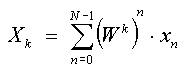The frequency of interest is located at term k.

`  f  =  k/N  fsample `

The weighting factors in the DFT equation are complex values obtained by sampling the cosine and sine functions. These complex numbers have the property that indexing them in the reverse direction generates the complex conjugates; the negative powers also produce complex conjugates. Doing both results in the original weighting factors, only with a different way of thinking about how they are generated.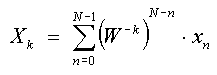In something of a role reversal, we can treat the powers of W in the DFT summation as the power terms in a polynomial, with the x terms as the multiplier coefficients. An efficient way to evaluate this polynomial is the nested form.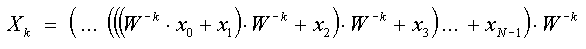As the nested form is evaluated term by term, it produces a sequence of intermediate results y:

```  y-1  = 0
y0   = W-k y-1  + x0
y1   = W-k y0   + x1
...
yN-1 = W-k yN-2 + xN-1
yN   = W-k yN-1
```

The final result yN equals the desired DFT sum. The N evaluation steps can be summarized by an iteration

```  yn   = W-k yn-1 + xn

n = 0, ... , N-1
```

While simple, this is still no more efficient than the original DFT.

The iteration equation has an equivalent transfer function in terms of discrete domain variable z.Multiplying top and bottom by the conjugate of the denominator terms, and incorporating the multiply operation that follows the final step of the nested sequence, we get the final Goertzel filter equation.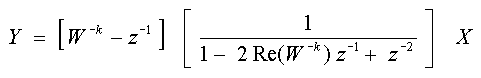Let us review the minor miracles.

1. The filtering is split into two simple cascaded parts.
2. The part of the filtering applied directly to the input sequence uses only real numbers.
3. One of the filter multipliers has reduced to a constant value of 1.0, eliminating half of the multiply operations.
4. The other multiplier does not change. It is not necessary to use a table of pre-computed coefficients as in an FFT or DFT.
5. The only value of y we really care about is the final one, yN. The second filter stage is a two-term FIR filter evaluated just once.

## Coding the Goertzel Algorithm

For applications with a real-valued measurement stream, the general complex-valued computations reduce to real-valued computations, and the coding can be implemented like this:

```realW = 2.0*cos(2.0*pi*k/N);
imagW = sin(2.0*pi*k/N);

d1 = 0.0;
d2 = 0.0;
for (n=0; n<N; ++n)
{
y  = x(n) + realW*d1 - d2;
d2 = d1;
d1 = y;
}
resultr = 0.5*realW*d1 - d2;
resulti = imagW*d1;
```

The results are the real and imaginary parts of the DFT transform for frequency k.

## Adjusting Filter Discrimination and Rejection

Now the point of this note: using the filter more effectively. Remember, Goertzel filtering is just a DFT in disguise, and an FFT is just a DFT applied to a lot of frequencies. Any selectivity problems that an FFT has, and any solutions that work for an FFT, will also apply to Goertzel filtering.

We know from studies of FFT's that each bin in an FFT is like a very sharply tuned filter. If a frequency is present within that filter's narrow range, the filter responds sharply. If the frequency drifts one step away, the filter response drops all the way to zero, while the response of the filter for the next bin increases to its peak. That's very good. But the problem is, what happens after that? And the answer is, a lot of bad things can happen.

You may have seen a plot like the following. This plot shows the magnitude response of one FFT filter over a frequency range.It is very clear that the filter responds to many frequencies that fall into the cracks between the N tuned filters in the array. The "rolloff envelope" has a shape that tapers away very slowly. All of which boils down to one thing: your FFT / DFT / Goertzel filter can respond to these spurious frequencies or combinations of them to generate "false alarms."

The solution to this is the same as it is for FFT analysis. By sacrificing some frequency resolution near the tuned frequency — that is, the filter peak is slightly more spread — it is possible to obtain greatly improved rejection of out-of-band frequencies. How is that done? Use a window.

As a quick review, a window for an FFT is a sequence of multipliers applied term-by-term to modulate the input data sequence. Windowing widens the response band of the filtering, but increases out-of-band rejection. Two common window sequences that are very effective:

Hamming Window

Terms are generated using the formula

` 0.54 - 0.46 * cos(2*pi*n/N)`

Pass band spans primary 2 bins plus first neighbors on each side.
Out of band rejection minimum -43 dB.

Exact Blackman

Terms are generated using the formula

` 0.426591 - .496561*cos(2*pi*n/N) +.076848*cos(4*pi*n/N)`

Pass band spans primary 2 bins plus first 2 neighbors on each side.
Out of band rejection minimum -66 dB.

For the selected window type, pre-compute the window terms and scale the input values as they arrive.

To get an idea of how much difference this makes, the following is the Goertzel filter's response when used in combination with a Hamming window.## Design Example

For a 50 Hz 3-phase power system, the system is supposed to be balanced, so the sum of the three voltage phases should be zero – a virtual ground. However, if there is a power system imbalance, the sum voltage will show a distinct 50 Hz component. The problem is to detect that frequency.

A local fault will not shift the frequency of the power grid by much. Presume that the frequency remains in the range 49 Hz to 51 Hz. A factor of 100 out-of-band rejection is judged to be adequate, so a Hamming window is selected for the filtering. When using a Hamming window, the Goertzel filter passband will span 4 frequency steps of the DFT.

Suppose that the sampling rate is 200 Hz. If 4 frequency steps cover 2 Hz, the entire 200 Hz band is covered in 400 steps. The 50 Hz center frequency is at 1/4 of the sampling frequency, so its frequency location is k=100. The Goertzel filter is now completely determined.

```realW = 2.0*cos(2.0*pi*100/400);
imagW = sin(2.0*pi*100/400);
```

Pre-compute the Hamming window terms. Apply it term by term to the input values as they arrive. Immediately apply the Goertzel filter.

```   for (n=0; n<400; ++n)
{
// Get new sample
...
x *= Hamm(n);

// Apply Goertzel filter
...
}
```

A new frequency detection result is produced after each 400 new samples, every 2 seconds.

## Demo

To demonstrate the effectiveness of the modified Goertzel filter for detecting a tone and rejecting out-of-band frequencies, an implementation of the windowed Goertzel filter is provided in the `GOERTZ.DLM` downloadable command module.

The download also contains a demo application for DAPstudio. The demo compares the operation of identical `GOERTZEL ` detection commands on two signals. The two signals are the same, except in one of them the 50 Hz content is suppressed using a very narrow band notch filter. The 50 Hz signal energy varies greatly in the randomized signal, but the `GOERTZEL ` detector has no difficulty seeing it. For the signal with the 50 Hz content removed, there is almost no response at all; clearly the `GOERTZEL ` detector rejects out of band frequencies very well.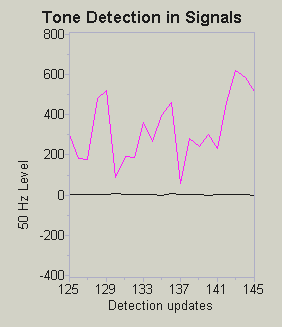## Conclusion

FFT and related methods such as Goertzel filtering process blocks of data to yield each result. For an FFT, all data for a block must be collected before any processing can begin. For a DFT or Goertzel filter, data are processed sequentially and efficiently as they arrive. When the end of the sequence is reached, the result is ready for issue.

This strategy for frequency detection still is subject to latency, because no result is available until the last sample of a block is received. Still, this approach can be useful in many detection and indirect control applications.

FFT's are notorious number crunchers. Even though they are fast on Data Acquisition Processor boards, fast is relative to how much CPU power you can afford to use. Compared to an FFT, the Goertzel algorithm is simple and much more efficient for detecting a single tone. With careful attention to the length of your filter (to adjust frequency discrimination) and with judicious use of windowing techniques (to suppress out-of-band effects), a Goertzel filter processing command can be a handy tool for your signal processing toolbox.